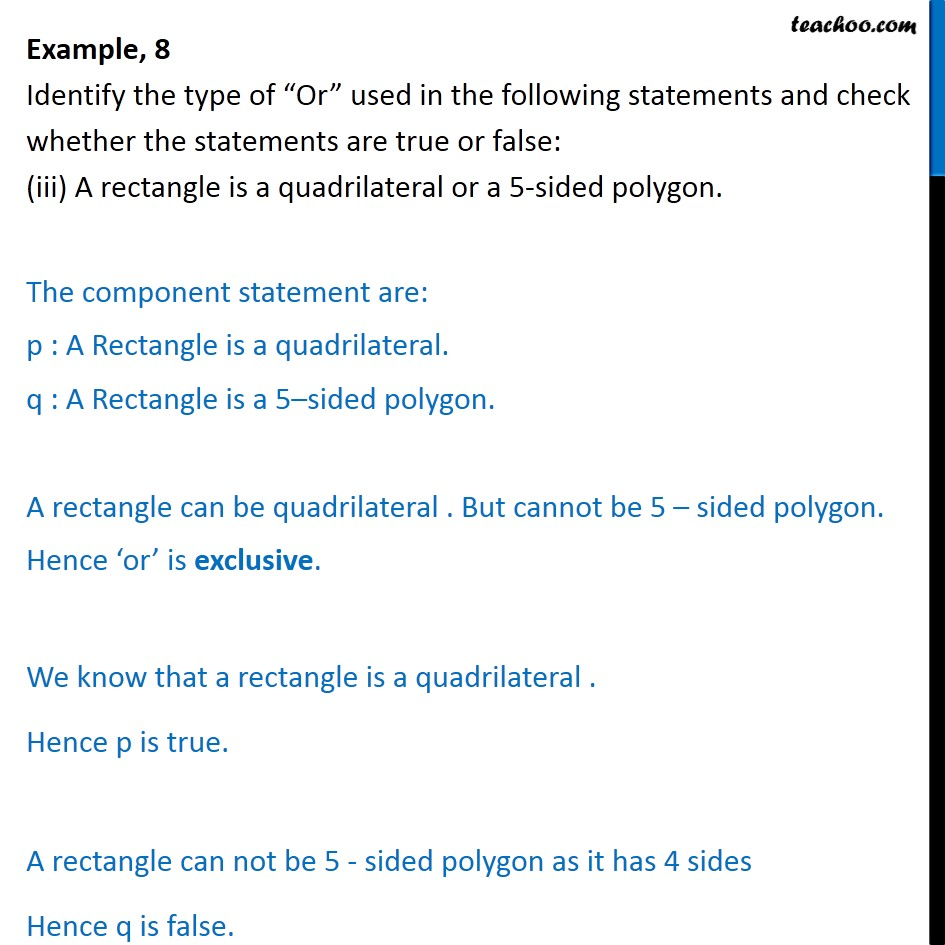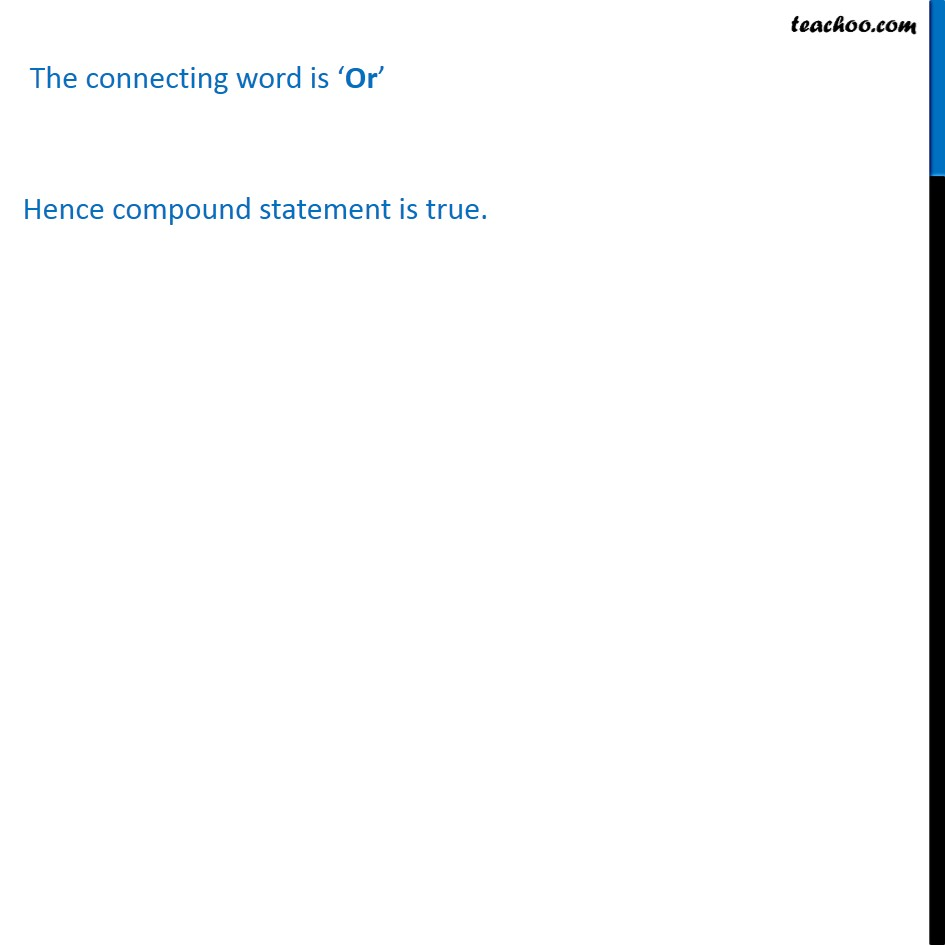Examples

Mathematical Reasoning
Serial order wiseLearn in your speed, with individual attention - Teachoo Maths 1-on-1 Class

### Transcript

Example, 8 Identify the type of “Or” used in the following statements and check whether the statements are true or false: (iii) A rectangle is a quadrilateral or a 5-sided polygon. The component statement are: p : A Rectangle is a quadrilateral. q : A Rectangle is a 5–sided polygon. A rectangle can be quadrilateral . But cannot be 5 – sided polygon. Hence ‘or’ is exclusive. We know that a rectangle is a quadrilateral . Hence p is true. A rectangle can not be 5 - sided polygon as it has 4 sides Hence q is false. The connecting word is ‘Or’ Hence compound statement is true.